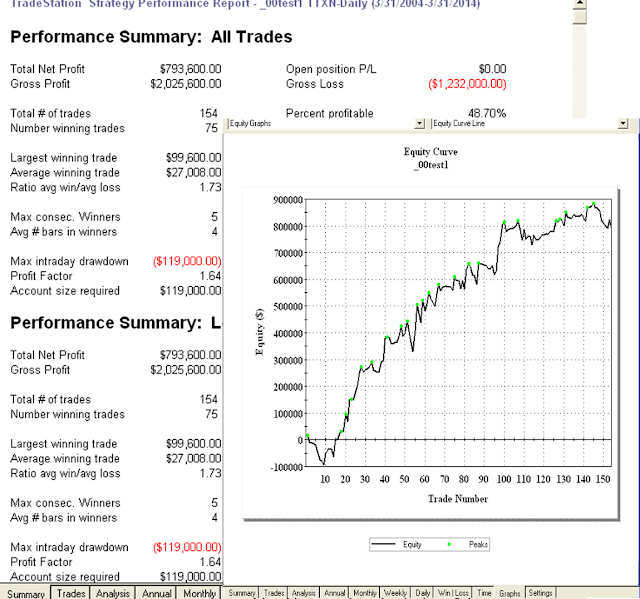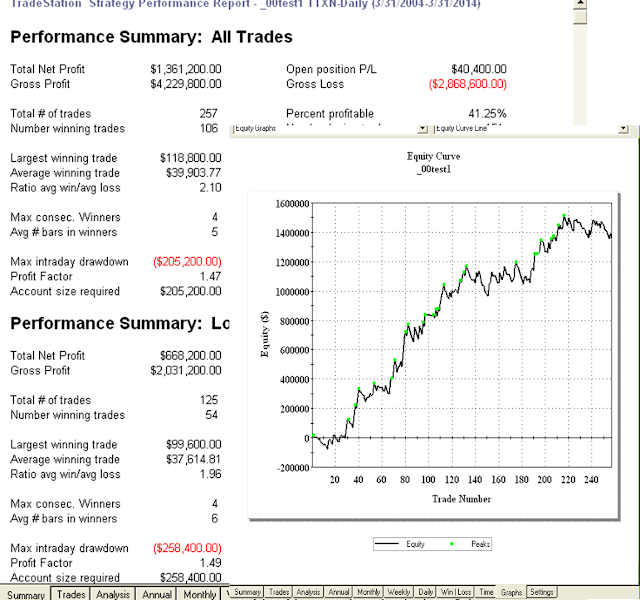## 2014年4月21日 星期一

### 指標組合 RSI + ADX [程式碼]

Long Entries:
The 20-bar moving average must be rising.
If the ADX is rising (ADX today is 0.20 or more higher than yesterday) then buy if the 14 bar RSI is less than 85.

If the ADX is not rising (ADX today is not 0.20 higher than yesterday) then buy if the 14 bar RSI is less than 50. Here is where you can influence the frequency of trading. For more trades use a higher threshold like 60. For fewer trades use a lower threshold like 40.
Long Exits
If the ADX is not rising (ADX today is not 0.20 higher than yesterday) then sell (long exit) if the 9-bar RSI is greater than 75.
If the ADX is rising (ADX today is 0.20 or more higher than yesterday) and the open profit is greater than (pick some amount - maybe 4 ATRs or some unit of price) then sell if the 9-bar RSI is greater than 75.
You need some additional exit rule for the losing trades. Use your favorite loss-limiting exit or you might want to exit when the price goes below the 20-dat moving average or when the 20-day moving average turns down. (See entry rule 1.)

if Average(Close ,20) > Average(Close,20) then Begin
if ( ADX(14)-ADX(14) ) > 0.2 and RSI(Close,14) < 85 then Buy next bar at market ;
and RSI(Close,14) < 50 then Buy next bar at market ;
end;

then ExitLong next bar at market ;
then ExitLong next bar at EntryPrice + TrueRange * 4 stop ;

if Average(Close ,20) < Average(Close,20) then ExitLong next bar at Average(Close ,20) stop ;if Average(Close ,20) < Average(Close,20) then Begin
if ( ADX(14)-ADX(14) ) > 0.2 and RSI(Close,14) > 15 then Buy next bar at market ;
and RSI(Close,14) > 50 then Buy next bar at market ;
end;

then ExitLong next bar at market ;

then ExitLong next bar at EntryPrice - TrueRange * 4 stop ;

if Average(Close ,20) > Average(Close,20) then ExitLong next bar at Average(Close ,20) stop ;